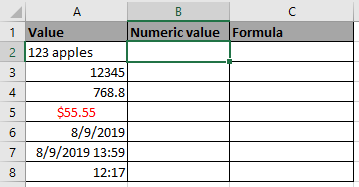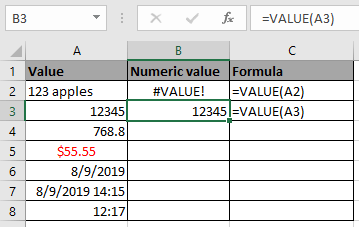# How to use the Excel VALUE function

The VALUE function is an Excel conversion function. It converts text(date, time & currency) to a numeric value.
Syntax:

=VALUE (text)

Text: text could be date, time or currency

Note: It returns #value! error if any text (alphabets) occurs

Let’s understand this function using it an example.
Here we have different values and we will apply the same formula to the values to get the result.Use the formula

=VALUE(A2)Here as you can see value function returns the #value! error where alphanumeric value occurs.The VALUE function returns the numeric values corresponding to the text.

Hope you understood how to use VALUE function and referring cell in Excel. Explore more articles on Excel conversion function here. Please feel free to state your query or feedback for the above article.

Related Articles:

How to Use TEXT Function in Excel

The CHAR Function in Excel

How to use CODE Function in Excel

Popular Articles:

How to use the VLOOKUP Function in Excel

How to use the COUNTIF function in Excel 2016

How to Use SUMIF Function in Excel

Terms and Conditions of use

The applications/code on this site are distributed as is and without warranties or liability. In no event shall the owner of the copyrights, or the authors of the applications/code be liable for any loss of profit, any problems or any damage resulting from the use or evaluation of the applications/code.